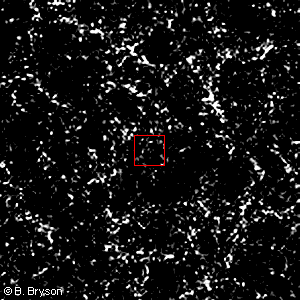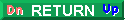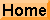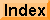1025 meters# More at 10 yottameters

Why is 1025 the largest view that we can represent with accuracy? All of the main images in this site represent a 30 degree field of view. Applying some basic trigonometry (the exercise is left to the student...), we can show that the one meter picture was taken from a height of a little less than 2 meters. Likewise, the 1 thousand million (109) light year image is seen from a distance of 2 thousand million light years. So if we take another step backward (make that a giant step) we will have stepped out to 20 thousand million light years -- outside our universe! I'm sorry, but I don't know what that would look like....

Does it make sense to ask questions like "How big is the universe?", or "How many quarks does the universe contain?" Well, yes it does. The universe must have a finite volume -- it has been expanding from the Big Bang at a certain rate for a certain time so the volume is large but calculable. On the other hand, the universe is all there is. There is no 'outside' -- there's no THERE there (as far as science can tell). As the universe continues to expand the volume it occupies just increases. Mathematically, the universe is finite but unbounded.

The question of the number of particles in the universe provides a more concrete answer. Say there are 1012 galaxies each containing 1012 stars each with a mass equal to the Sun. The Sun has a large but finite number of atoms. The mass of the Sun is about 1031 kilograms. From basic chemistry a kilogram of hydrogen contains about 1027 atoms. If you multiply it all out using these numbers you get about 1082 particles in the universe -- a (very) large but finite number. Say that I have underestimated the number of galaxies. Perhaps there are 1024 galaxies in the universe. That would add another 12 zeros. The point is that it is still finite and calculable. There is a number in mathematics called a googol -- defined as 10100. That's a 1 followed by 100 zeros. It would appear that the universe contains less than a googol of quarks.Copyright © 2016 by Bruce Bryson